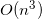# Arbitrary Precision Iterative Solver – GMRES

by on October 11, 2011

The multiprecision toolbox provides all the common routines to do the matrix computations with arbitrary precision – solvers, decompositions, eigensolvers, matrix functions, etc. Recently we have made important addition – iterative methods for large/sparse matrices.

Direct solvers are designed to find solution in finite number of steps (e.g. Gauss elimination). Such methods rely heavily on `BLAS3` operations (matrix-matrix, `xGEMM`) and hence complexity is. Naturally this limits applicability of the methods for large matrices, as complexity might be prohibitive.

Iterative methods avoid cubic complexity growth by using only the matrix-vector multiplications (`BLAS2 - xGEMV` or `SpMV`). Thus they make solving large dense and sparse matrix possible.

However this comes with the price of slow convergence, loosing orthogonality of subspace vectors, etc. Some of the issues can be resolved by preconditioners and re-orthogonalizations which in turn increase complexity of the algorithm.

Extended accuracy allows studying the effects and actually can speed-up the solver by allowing it to converge in less number of steps or avoiding re-ortrhogonalization (as there is no loss of orthogonality). Our user manual has good example on how convergence speed changes with precision.

Toolbox includes following iterative solvers: `BiCG, BiCGSTAB, BiCGSTABL, CGS, GMRES, MINRES` and `PCG`. All methods are capable of working with arbitrary precision sparse and dense matrices. Routines are implemented in different variants in order to enable drop-in replacement for MATLAB’s equivalent built-in functions.

For example, GMRES supports various input types (matrix and function handle) and preconditioners:

`x = gmres(A,b) gmres(A,b,restart) gmres(A,b,restart,tol) gmres(A,b,restart,tol,maxit) gmres(A,b,restart,tol,maxit,M) gmres(A,b,restart,tol,maxit,M1,M2) gmres(A,b,restart,tol,maxit,M1,M2,x0) [x,flag] = gmres(A,b,...) [x,flag,relres] = gmres(A,b,...) [x,flag,relres,iter] = gmres(A,b,...) [x,flag,relres,iter,resvec] = gmres(A,b,...)`

In this post we want to show how multiprecision GMRES can be used in various situations and set of parameters.

### Matrix Input

Create sparse matrix and assume that solution is all `1`‘s:

```A = sparse(gallery('wilk',21)); b = sum(A,2); maxit = 50; M1 = diag([10:-1:1 1 1:10]);```

Then solve using quadruple precision machine epsilon as a tolerance (`~1.9e-34`):

```>> x = gmres(mp(A),mp(b),10,mp('eps'),maxit,mp(M1));   'gmres(10) converged at outer iteration 6 (inner iteration 2) to' 'a solution with relative residual 4.9e-35.'```

### Function Handle

We can use functions for matrix-vector product and preconditioner instead of plain matrices.

```function x1 = gmres_fun   n = 21; b = afun(mp(ones(n,1))); maxit = 15; x1 = gmres(@afun,b,10,mp('eps'),maxit,@mfun);   function y = afun(x) y = [0; x(1:n-1)] + ... [((n-1)/2:-1:0)'; (1:(n-1)/2)'].*x + ... [x(2:n); 0]; end   function y = mfun(r) y = r ./ [((n-1)/2:-1:1)'; 1; (1:(n-1)/2)']; end end```

Result are the same:

```>> x = gmres_fun;   'gmres(10) converged at outer iteration 6 (inner iteration 2) to' 'a solution with relative residual 4.9e-35.'```

### Preconditioner

This example demonstrates the use of gmres with preconditioner (no restarts) in quadruple precision.

```load west0479; A = west0479; b = full(sum(A,2));   tol = mp('1e-30'); maxit = 50;   [x0,fl0,rr0,it0,rv0] = gmres(mp(A),mp(b),[],tol,maxit);   figure; semilogy(0:maxit,rv0/norm(b),'-o'); xlabel('Iteration number'); ylabel('Relative residual'); title('GMRES');   % Compute ILUTP in double precision [L,U] = ilu(A,struct('type','ilutp','droptol',1e-6)); [x1,fl1,rr1,it1,rv1] = gmres(mp(A),mp(b),[],tol,maxit,mp(L),mp(U));   figure; semilogy(0:it1(2),rv1/norm(b),'-o'); xlabel('Iteration number'); ylabel('Relative residual'); title('GMRES + ILUTP Preconditioner');```Important note. It is not necessary for preconditioner to have high accuracy and we can use `double` precision to save time.